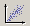# Incident angle of each pixel of Sentinel - 1A

1. How to obtain the incident angle of each pixel of Sentinel - 1A
If you are processing data in SNAP, how do you get the corrected angle file?

2. How to use the tool Band _ Math to generate backscattered images from different angles so as to analyze the relationship between angles and backscattered images in the back lane.

1 Like

regarding the angle of each pixel: Local incidence angle (SAR grid)
regarding the relationship between incidence angle and backscatter you can use the scatter plot or the correlative plot view:

1 Like

What can we understand from this plot?

how backscatter changes over the image and according to the incidence angle. If you are interested in backscatter analyses but your areas of interest lie at different slopes, this helps you estimate how the analysis is biased.

can we get coefficient of correlation and trend line in scatter plot

yes, you need to import points with numerical values (as csv or shp) and then use the correlative plot viewBut I want a correlation between local incidence angle and backscratching coefficient raster image

if you want to have the coefficient and a trend line in SNAP, you need one point data set. It is probably easier to export selected values of both rasters as a table and have the trend line generated in MS Excel, for instance.

1 Like

alright thank you

Mr @ABraun how can I export all pixel values in a subset image or Specified area to analysis in Excel?

please look here: Export pixel values of a whole region

1 Like

thanks a lot for your support.

you’re welcome. This forum contains a lot of answers, but feel free to let us know when the solutions offered in the topics I link don’t match your requirements.

1 Like